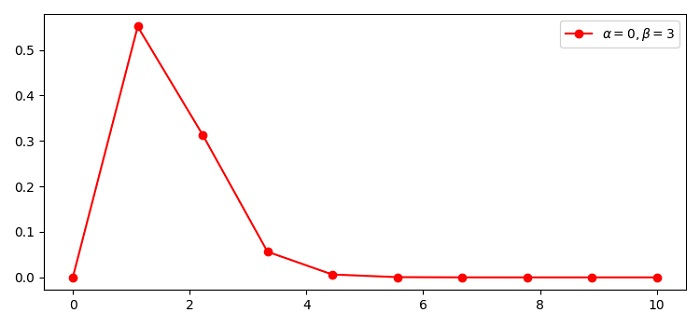# How to plot gamma distribution with alpha and beta parameters in Python using Matplotlib?

To plot gamma distribution with alpha and beta parameters in Python, we can use gamma.pdf() function.

## Steps

• Set the figure size and adjust the padding between and around the subplots.

• Create x using numpy and y using gamma.pdf() function at x of the given RV.

• Plot x and y data points using plot() method.

• Use legend() method to place the legend elements for the plot.

• To display the figure, use show() method.

## Example

import numpy as np
import scipy.stats as stats
from matplotlib import pyplot as plt

plt.rcParams["figure.figsize"] = [7.50, 3.50]
plt.rcParams["figure.autolayout"] = True

x = np.linspace(0, 10, 10)
y = stats.gamma.pdf(x, a=5, scale=0.333)

plt.plot(x, y, "ro-", label=(r'$\alpha=0, \beta=3$'))
plt.legend(loc='upper right')

plt.show()

## Output